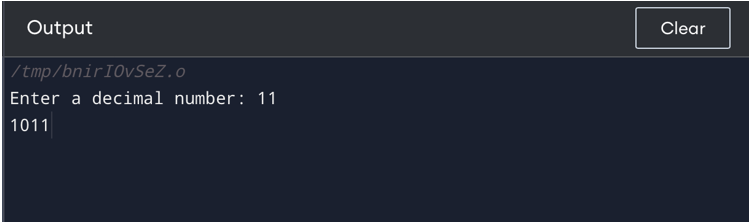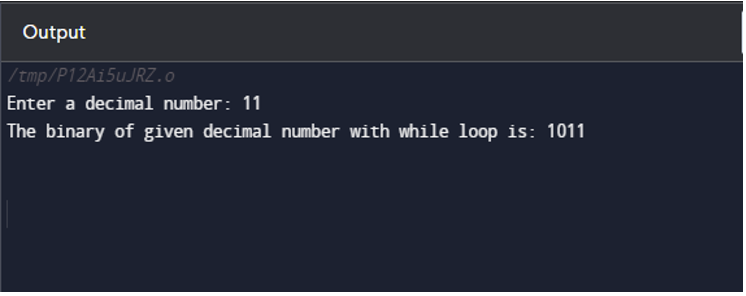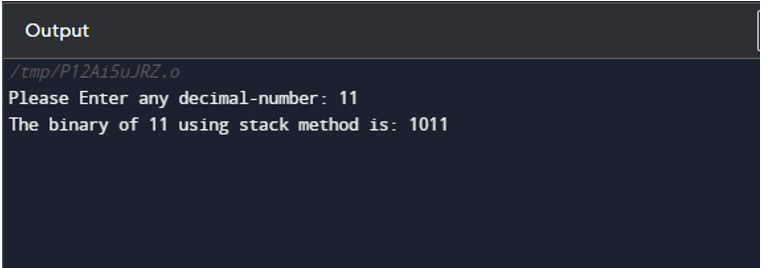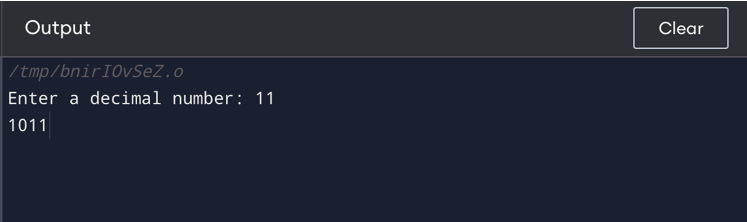| by Arround The Web | No comments

## How to Convert Decimal to Binary in C

Computer systems frequently employ binary numbers to represent data and instructions, but since decimal digits are simpler for people to understand than binary hence, conversion is required. This article will go through the understanding between decimals into binary and provide program examples of different methods that convert decimals into binary in the language of programming called C.

## How to Convert Decimal to Binary in C?

The given decimal value is divided by 2 several times, with the remainder being recorded until we get to 0 as the final quotient. This process is used to convert integers from decimal to binary. The formula that demonstrates the conversion process from decimal to binary is regarded to be the stages that are as follows:

Step 1: Find the remainder by dividing the number by 2, then add it to an array.

Step 2: Next, divide the remainder by two.

Step 3: Carry out the first two steps again until the result exceeds 0.

Step 4: Display the array backward to obtain the number’s binary form. The Least-Significant-Bit (LSB) of a binary number lies at the top, whereas the Most-Significant-Bit (MSB) is towards the bottom, which is another way to interpret this. The binary equivalent of the provided decimal number is this one.

To grasp this better, here is a conversion of  1110 in decimal to binary conversion:

 Division by 2 Quotient Remainder 11 ÷ 2 5 1 (LSB) 5 ÷ 2 2 1 2 ÷ 2 1 0 1 ÷ 2 0 1 (MSB)

Now, write the remainder in the reverse order, hence, decimal (1110) becomes (10112).

The remainder will be noted and then written so that the (MSB) of the binary number comes first, then the rest. Consequently, 10112 is the binary equivalent of the given decimal value 1110. As a result, 1110 = 10112. Following is the implementation of the above-mentioned decimal into binary conversion in C language.

## Methods Used to Convert Decimal to Binary

These methods that are used in C language convert decimal digits into binary:

Now, implement the conversion of decimals into binary ones by one by the above-mentioned approaches.

## Method 1: Decimal to Binary in C Programming with for Loop

Below is the conversion of the decimal digit(11) into binary with the help of for-loop in C:

#include <stdio.h>
void convert(int num1) {
if (num1 == 0) {
printf("0");
return;
}
int binary_num; // Assuming 32 bit integer.
int i=0;
for ( ;num1 > 0; ){
binary_num[i++] = num1 % 2;
num1 /= 2;
}
for (int j = i-1; j >= 0; j--)
printf("%d", binary_num[j]);
}
int main() {
int num1;
printf("Enter a decimal number: ");
scanf("%d", &num1);
convert(num1);
return 0;
}

The above program is using the for loop to convert the decimal number provided by the user to binary. The output is:## Method 2: Decimal to Binary in C Programming with while Loop

In this method, the while-loop in C is used to convert the decimal digit (11), into binary, as shown below:

#include <stdio.h>
int main() {
int decimal_num, binary_num = 0, base = 1, remainder;
printf("Enter a decimal number: ");
scanf("%d", &decimal_num);
while(decimal_num > 0) {
remainder = decimal_num % 2;
binary_num = binary_num + remainder * base;
decimal_num = decimal_num / 2;
base = base * 10;
}
printf("The binary of given decimal number with while loop is: %d", binary_num);
return 0;
}

The four integer variables decimal_num, binary_num, base, and remainder are first declared in this program. The user enters a decimal_num, which we will convert to its binary_num. The binary conversion is carried out using the while loop.## Method 3: Decimal to Binary in C Programming with Stack

This is the straightforward method with a stack-based C program that changes a decimal value to a binary:

#include <stdio.h>

#define MAX_SIZE 32 // maximum number of bits in binary representation
int main() {
int decimal_num, binary[MAX_SIZE], top = -1;
scanf("%d", &decimal_num);
while (decimal_num > 0) {
binary[++top] = decimal_num % 2;
decimal_num /= 2;
}
printf("The binary of %d using stack method is: ");
while (top >= 0) {
printf("%d", binary[top--]);
}
return 0;
}

We first declare the three integer variables top, binary, and decimal_num in this program. The top is the index of the highest element in the stack, decimal is the number in a decimal form that is entered by the user, binary is an array that will contain the bits in binary as MAX_SIZE bits and decimal_num is the binary array. After that, the binary conversion is carried out using a while loop.

The output is:## Method 4: Decimal to Binary in C Programming with Bitwise Operator

An operator known as a bitwise operation manipulates each of the bits of binary symbols that represent integers. The following is a basic C script that uses bitwise operations to translate a number in decimals into binary:

#include <stdio.h>

//This method will show all the 4 bits of a number
void conversion(int num1) {
// Consider a 4-bit number
for (int i = 3; i >= 0; i--) {
int bit = (1 << i);
if (num1 & bit)
printf("1");
else
printf("0");
}
}
int main() {
int num1;
printf("Enter a decimal number: ");
scanf("%d", &num1);
conversion(num1);
return 0;
}

This program will execute a for loop through the number starting at the most significant bit and ending at the bit with the least significance. By performing a “bitwise AND” operation on mask and num, we may determine if the bit is either 0 or 1. If it is non-zero, the present bit is 1; otherwise, it is 0.

To show the binary form of num1, output the bit after each iteration. The final output after complete execution will be as below:## Conclusion

It is an essential skill in computer programming to convert numbers from decimal to binary. Transforming decimal numbers to binary enables us to carry out different operations in C programming. This article provided 4 methods to convert decimal values into binary values.

Source: linuxhint.com